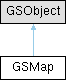OpenTTD GameScript API  20220624-master-gffd3c11ba7
GSMap Class Reference

Class that handles all map related functions. More...

Inheritance diagram for GSMap:## Static Public Member Functions

static bool IsValidTile (TileIndex tile)
Checks whether the given tile is valid. More...

static TileIndex GetMapSize ()
Gets the number of tiles in the map. More...

static uint32 GetMapSizeX ()
Gets the amount of tiles along the SW and NE border. More...

static uint32 GetMapSizeY ()
Gets the amount of tiles along the SE and NW border. More...

static int32 GetTileX (TileIndex tile)
Gets the place along the SW/NE border (X-value). More...

static int32 GetTileY (TileIndex tile)
Gets the place along the SE/NW border (Y-value). More...

static TileIndex GetTileIndex (uint32 x, uint32 y)
Gets the TileIndex given a x,y-coordinate. More...

static int32 DistanceManhattan (TileIndex tile_from, TileIndex tile_to)
Calculates the Manhattan distance; the difference of the X and Y added together. More...

static int32 DistanceMax (TileIndex tile_from, TileIndex tile_to)
Calculates the distance between two tiles via 1D calculation. More...

static int32 DistanceSquare (TileIndex tile_from, TileIndex tile_to)
The squared distance between the two tiles. More...

static int32 DistanceFromEdge (TileIndex tile)
Calculates the shortest distance to the edge. More...

## Static Public Attributes

static const int TILE_INVALID = (int)INVALID_TILE
Invalid TileIndex.

## Detailed Description

Class that handles all map related functions.

## ◆ DistanceFromEdge()

 static int32 GSMap::DistanceFromEdge ( TileIndex tile )
static

Calculates the shortest distance to the edge.

Parameters
 tile From where the distance has to be calculated.
Precondition
IsValidTile(tile).
Returns
The distances to the closest edge.

## ◆ DistanceManhattan()

 static int32 GSMap::DistanceManhattan ( TileIndex tile_from, TileIndex tile_to )
static

Calculates the Manhattan distance; the difference of the X and Y added together.

Parameters
 tile_from The start tile. tile_to The destination tile.
Precondition
IsValidTile(tile_from).
IsValidTile(tile_to).
Returns
The Manhattan distance between the tiles.

## ◆ DistanceMax()

 static int32 GSMap::DistanceMax ( TileIndex tile_from, TileIndex tile_to )
static

Calculates the distance between two tiles via 1D calculation.

This means the distance between X or the distance between Y, depending on which one is bigger.

Parameters
 tile_from The start tile. tile_to The destination tile.
Precondition
IsValidTile(tile_from).
IsValidTile(tile_to).
Returns
The maximum distance between the tiles.

## ◆ DistanceSquare()

 static int32 GSMap::DistanceSquare ( TileIndex tile_from, TileIndex tile_to )
static

The squared distance between the two tiles.

This is the distance is the length of the shortest straight line between both points.

Parameters
 tile_from The start tile. tile_to The destination tile.
Precondition
IsValidTile(tile_from).
IsValidTile(tile_to).
Returns
The squared distance between the tiles.

## ◆ GetMapSize()

 static TileIndex GSMap::GetMapSize ( )
static

Gets the number of tiles in the map.

Returns
The size of the map in tiles.
Postcondition
Return value is always positive.

## ◆ GetMapSizeX()

 static uint32 GSMap::GetMapSizeX ( )
static

Gets the amount of tiles along the SW and NE border.

Returns
The length along the SW and NE borders.
Postcondition
Return value is always positive.

## ◆ GetMapSizeY()

 static uint32 GSMap::GetMapSizeY ( )
static

Gets the amount of tiles along the SE and NW border.

Returns
The length along the SE and NW borders.
Postcondition
Return value is always positive.

## ◆ GetTileIndex()

 static TileIndex GSMap::GetTileIndex ( uint32 x, uint32 y )
static

Gets the TileIndex given a x,y-coordinate.

Parameters
 x The X coordinate. y The Y coordinate.
Precondition
x < GetMapSizeX().
y < GetMapSizeY().
Returns
The TileIndex for the given (x,y) coordinate.

## ◆ GetTileX()

 static int32 GSMap::GetTileX ( TileIndex tile )
static

Gets the place along the SW/NE border (X-value).

Parameters
 tile The tile to get the X-value of.
Precondition
IsValidTile(tile).
Returns
The X-value.
Postcondition
Return value is always lower than GetMapSizeX().

## ◆ GetTileY()

 static int32 GSMap::GetTileY ( TileIndex tile )
static

Gets the place along the SE/NW border (Y-value).

Parameters
 tile The tile to get the Y-value of.
Precondition
IsValidTile(tile).
Returns
The Y-value.
Postcondition
Return value is always lower than GetMapSizeY().

## ◆ IsValidTile()

 static bool GSMap::IsValidTile ( TileIndex tile )
static

Checks whether the given tile is valid.

Parameters
 tile The tile to check.
Returns
True is the tile it within the boundaries of the map.